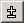Productions: Term and Factor
 Examples > Calculator > Productions: Term and Factor   The Term-Production is constructed analogously to the Expression production; multiplication and division take the places of the addition and subtraction:   {{double d;}} Factor[d] {{xd = d;}} (  "*" Factor[d] {{xd *= d;}}  |"/" Factor[d] {{ if(d == 0)   throw CTT_Error("Division by null"); xd /= d; }}   )*     If the denominator is null, the program is stopped by a call of:   throw CTT_Error("Division by null");   The text "Division by null" then will be shown in the log window..     The production Factor is:   {{ bool bPlus = true; double d; }} ( "-" {{bPlus = false;}} )? (   Number[d] | "(" Expression[d] ")" ) {{ if(bPlus) xd = d; else xd = -d; }}    It can be shown without actions by clicking on the collapse code button:( "-" )? ( Number | "(" Expression ")"  )   Parenthesis followed by a question mark characterizes the included expression as optional, i.e., it may occur in the text or not. So a Factor is an optional minus sign followed by either a number or a bracket. Here the parser becomes reflexive. The Expression production had called the Term production, which called the Factor production. Inside the Factor production the Expression production can be called again. This reflexivity mirrors the possible nesting of parenthesis inside of arithmetic problems, e.g.  "3 + ((3.2 + 8.9 - 4.6) * 5.6)"   The minus sign is optional. If it exists or not in the text, will be memorized in a boolean variable. Variables of the type bool can have the value true or false. The variable bPlus is declared at the beginning of the Factor production and set to the value true:   bool bPlus = true;   If the minus sign is found at the actual text position, this value will be set to false.  After the evaluation of the Number production or of a bracket, the result will be negated or not, depending on the value of bPlus. For this are the instructions:   if(bPlus) xd = d; else xd = -d;   The structure   if ( ; ;   means, that if the condition "condition" is fulfilled, that means, it has the value true, the instruction "instruction1" will be executed otherwise "instruction2".